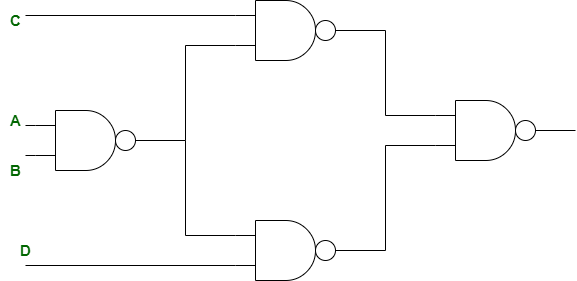GFG App
Open AppBrowser
Continue

# ISRO | ISRO CS 2020 | Question 60

Minimum number of NAND gates required to implement the following binary equation (A’+B’)(C+D)
(A) 4
(B) 5
(C) 3
(D) 6

Explanation: Given (A’+B’)(C+D),

According to NAND gate property,

```= (A'+B')(C+D)
= (A'+B')C + (A'+B')D
= (AB)'C + (AB)'D
= [{(AB)'C}'.{(AB)'D}']' ```Therefore, 4 NAND gates are required.

My Personal Notes arrow_drop_up